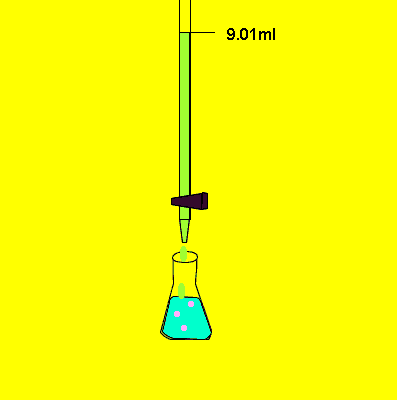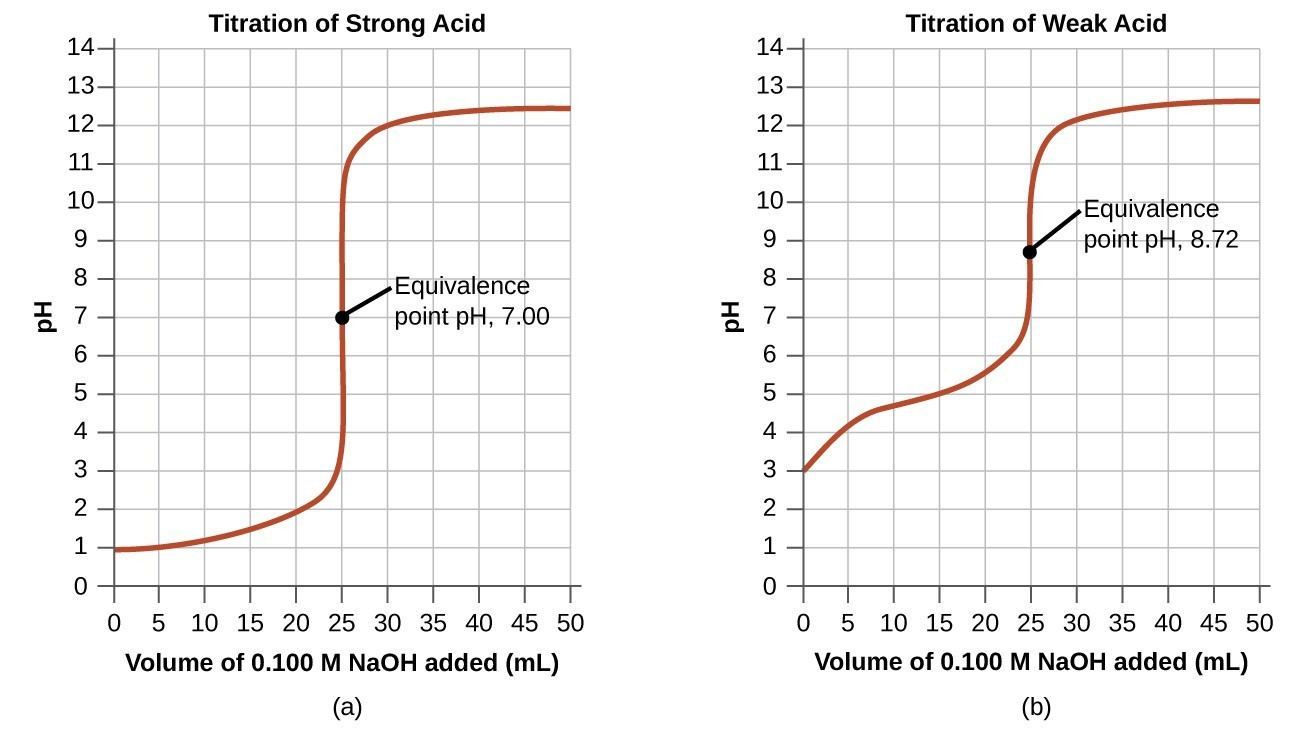Titration is a type of quantitative analysis which is used to determine the unknown concentration of a known analyte by using a solution of known concentration. Titration is a volumetric analysis as volume measurement plays a key role.

The word titration comes from the French word ‘titre‘ meaning “a measure of fitness or purity”. Volumetric analysis originated in the late 18th century.

Titration is one of the most used laboratory methods. It is a type of wet chemical analysis (classical method), sometimes also referred to as titrimetry.

Outline

## General procedure• Fill a titration flask with a calculated amount of analyte.
• Fill burette with a titrant.
• Add two to three drops of indicator in analyte solution.
• Note the initial level of liquid in the burette.
• Start adding titrant from burette slowly.
• Determine endpoint very carefully.
• When the color changes permanently, stop the flow from the burette.
• Note the final reading of liquid in the burette.
• Repeat this process two to three times for precise results.

### Conditions for titration

• There must be simple reaction which can easily be expressed by chemical equation.
• Substance to be determine should react completely with reagent.
• Reaction should be relatively fast (ionic reaction).
• There must be alternation of some physical or chemical properties of solution at the equivalence point.
• Suitable indicator should be available for end point determination.
• Indicators should give sharp end point detection.
• Reagent should be specific (only react with analyte).

## Types of titration

The following are the different types of titrations:

• Acid base titration
• Redox titration
• Complexometric titration
• Nonaqueous titration
• Back titration
• Precipitation titration
• Zeta potential titration
• Potentiometric titration
• Amperometric titration
• Spectrophometric titration

### 1. Acid base titration

Acid base titration is also known as neutralization titration. It is an analytical technique used to find unknown concentrations of acid or base by neutralizing them with a standard solution of acid or base. It depends upon the neutralization between acid and base when mixed in a solution.

In order to determine endpoint accurately, suitable pH indicators are used. For example, methyl orange, methyl red, phenolphthalein, thymol blue, phenol red, etc.

If both acid and base are strong electrolytes, then the endpoint will be neutral at pH 7.

If either acid or base is a weak electrolyte, the solution at equivalence point will be slightly alkaline or slightly acidic.

### 2. Redox titration

Redox titration is based on an oxidation reduction reaction between oxidizing and reducing agents. It is used to find out the concentration of analyte through redox reaction between analyte and titrant. In redox titrations, analyte and titrant are oxidizing or reducing agents. Redox indicators are used to determine the endpoint. For example, methyl blue, diphenylamine, phenosafranine, Diphenylamine sulphonic acid, Indigo-5,5-disulfonate, etc.

Some time no indicator is required due to the intense colors of constituents.

There are different types of redox titration:

• Permagnate titrations
• Dichromate titrations
• Iodometric titration
• Iodimetric titration

### 3. Complexometric titration

Complexometric titrations are used to quantification of metals by forming a colored complex. This is a type of volumetric analysis that is used to determine metal analytes in a solution by using suitable titrant and specific indicators.

Indicators of complexometric titrations are EBT, xylenol orange, calcein, murexide, thiocyanate, etc. Mostly EDTA is used as the titrant as it can form stable complexes with most of metals ions. It involves the formation of colored complex which is the endpoint of the complexometric titration.

### 4. Nonaqueous titration

In this type of titration, analyte is dissolved in a solvent that do not contain water. This is applied to weak acids or bases that give poor endpoints in aqueous solvents and also to those substances that are not soluble in water.

Nonaqueous solvents

The most commonly used nonaqueous solvents are glacial acetic acid, acetonitrile, dimethylformamide, alcohols, dioxan, etc.

Nonaqueous Indicators

The indicators used in non aqueous titrations are crystal violet, methyl red, thymol blue, 1-naphthol benzene.

### 5. Back titration

Back titration is also known as indirect titration. Here known excess amount of standard reagent is used to determine the concentration of an analyte. The remaining excess reagent is back titrated with the second reagent. So, this way the actual amount of titrant consumed by the analyte is measured.

Back titration are performed where there is difficulty in finding out endpoint in normal conditions or there is a slow reaction between titrant and analyte.

Many metals may precipitate or may form inert complexes in normal conditions. Then the excess of standard EDTA is added to the solution. The excess of EDTA is then back titrated with standard metal ion solutions (sulfate or chloride of Zn and Mg).

### 6. Precipitation titration

Precipitation titration involves the formation of precipitate during titration. When titrant is added to the solution containing analyte, it starts the formation of insoluble particles. It continues till the whole analyte is converted into precipitate (solid mass). The endpoint is determined by the formation of colored precipitates.

Adsorption indicators are also used in precipitation titrations which include eosin yellow, ferric alum, tartrazine, phenosaframine, dichlorofluroscein, etc. An example of precipitation titration is the determination of chloride ion by using silver nitrate.

### 7. Zeta potential titration

Zeta potential titration is used for heterogeneous solutions i.e colloids and emulsions, having high surface area. The endpoint is determined using zeta potential. No indicator is used. Zeta potential is the electrical potential of the colloidal system. It is used to determine isoelectric point by adding a surfactant or changing pH when surface charge becomes zero

### 8. Potentiometric titration

Potentionmetric titration is performed by using a potentiometer which detects the potential difference across pair of electrodes. It is especially useful in the case of colored or turbid solutions and for detecting the presence of unsuspected species in a solution. Simply dip a pair of electrodes in a sample solution and potentiometer will detect the change in potential when the titrant is added or concentration of ions is changed.

One of the electrodes is known as a reference electrode or counter electrode and the other one is known as an indicator or working electrode. Reference electrode has constant potential. Whereas, indicator electrodes respond to change in concentration of the analyte.

### 9. Amperometric titration

Amperometric titration involves the detection of electric current produced during titration. The current produced is proportional to the concentration of electroactive substances. Constant current is maintained with the potential of the indicator electrode. The endpoint is the change in current during titration. Special types of electrodes are used (rotating platinum electrode) for amperometric titrations.

### 10. Spectrophometric titration

Spectrophometric titrations are performed using a spectrophotometer. Here the endpoint is evaluated from the data on the absorbance of the solution in accordance with Beer’s Lambert Law. Such type of titrations are carried out in special titration cells.

A cell containing solution is placed in the light path and a specific wavelength is selected. The endpoint is determined graphically.

## Importance of titration

Titration is important in many ways:

• It is used to determine the concentration of the analyte.
• In pharmaceutical industry, quantification of many active pharmaceutical ingredients is done by titration.
• It is important in the food industry to determine the amount of reactant in a sample.
• Standardisation is done with the help of titration.
• It also helps in determination of organic functional groups (COOH, RCOOR).

There are many advantages of titration

• The precision is better than most instrumental methods.
• Instrumentation is very simple.
• It is a cheap method of analysis.
• The instruments does not require recalibration.
• It is a quick method of analysis.

There are many disadvantages of titration:

• This technique is less sensitive than instrumental analysis.
• It is time taking when a large number of similar analyses are performed.
• It is less selective than instrumental analysis
• Exact endpoints cannot be determined with titrations.
• The possibility of human error is high.

## Concepts Berg

Define standardization.

Standardization is a process that is used to determine the actual concentration of a solution of known concentration by titrating it with a primary standard.

Define primary standard?

A primary standard is a highly purified compound that serves as reference material in all volumetric analyses. Primary standards are used for the standardization of compounds. For example, sodium carbonate, silver nitrate, sodium tetraborate, sodium hydrogen phthalate, potassium bromate, potassium dichromate, iodine, benzoic acid, etc.

What are the features of the primary standard?

• It should be easily available.
• It should be inert.
• It should maintain its composition unchanged during storage.
• It should be cheap.
• It should be readily soluble under given conditions.
• It should be of large molar mass.

What is a secondary standard?

Secondary standards are substances whose concentrations are determined by comparing them against primary standards. Secondary standards can also be used for standardization purposes.

How is titration important in chemistry?

Titration is an important technique in chemistry as:

• It is used to determine the concentration of analyte in the sample.
• It is used for quantitative analysis of many drug substances.
• It is also used to find the amount of reactant in sample

What is a titration curve?

The titration curve is a graphical representation of the pH of a solution during the titration.

For example, titration curve of strong acid and base is:• In the beginning, pH is low and increases gradually as acid is neutralized by adding a base.
• For strong acid and base, the equivalence point is at pH 7.
• Beyond the equivalence point, pH increases slowly.
• After the equivalence point, the pH rise steeply.

What is the significance of blank titration?

Blank titration is performed to increase the accuracy of the results. They are performed when there are suspected impurities or contamination in the sample.

What are the advantages of potentiometric titrations?

There are many situations where potentiometric titration has advantages over classical indicator methods i.e

• When the solution is turbid or fluorescent.
• When the solution is colored (indicator masked).
• When there is no suitable indicator available for titration.
• For titration of mixtures of acids, bases, and halides

What is the application of precipitation titration?

• It is used for the determination of the concentration of metal ions in the sample solution.
• It is used to determine salt content in food and beverages.
• It is used for the determination of halide ions in solution.

Why is there importance of performing three or more trials in titration?

Titration is repeated 3 to 5 times to get accurate results by over coming human errors. Because there is possibility of error in endpoint determinition. Then,  average of results is calculated.

Why is controlling pH necessary in complexometric titration?

Control of pH is very important in complexometric titrations because H+ ions play important role in chelation. So the pH must be maintained (constant) during complexometric titration. And also certain indicators are pH dependent. They work at a specific pH.

What is the purpose of standardization in chemistry?

Standardization is performed to determine the exact concentration of that solution. It increases the reliability and accuracy of results.

What is the meaning of ‘residual titration?

Back titration is also known as residual titration. Back titration is performed by adding known excess amount of standard reagent to sample solution. The remaining excess reagent is back titrated with the second reagent. In this way, the actual amount of titrant consumed by the analyte is determined.